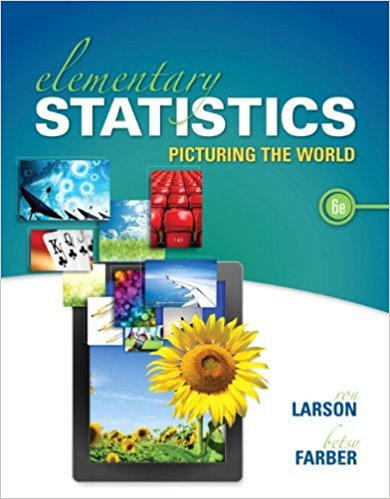# Using Technology to Find Quartiles and Draw Graphs In## Problem 25E Chapter 2.5

Elementary Statistics: Picturing the World | 6th Edition

• 2901 Step-by-step solutions solved by professors and subject experts
• Get 24/7 help from StudySoup virtual teaching assistantsElementary Statistics: Picturing the World | 6th Edition

4 5 0 432 Reviews
22
3
Problem 25E

Using Technology to Find Quartiles and Draw Graphs In Exercise, use technology to (a) find the data set’s first, second, and third quartiles, and (b) draw a box-and-whisker plot that represents the data set.

TV Viewing The numbers of hours of television watched per day by a sample of 28 people

 2 4 1 5 7 2 5 4 4 2 3 6 4 3 5 2 0 3 5 9 4 5 2 1 3 6 7 2
Step-by-Step Solution:

Step 1 of 3:

The data about the numbers of hours of television watched per day by a sample of 28 people is given.

 2 4 1 5 7 2 5 4 4 2 3 6 4 3 5 2 0 3 5 9 4 5 2 1 3 6 7 2
By using software we have to find the first, second, and third quartile for the given data set.We have to draw a box-and whisker plot that represent the data.

Step 2 of 3:

(a)

The median is the middle most value of the data set after arranging the data in an order (Q2).First quartile is the median of data points which is below the median (Q1).Third quartile is the median of data points which is above the median (Q3).

We can find the quartiles by using Minitab.Enter the given data in MinitabGo to StatSelect Basic StatisticChoose Display descriptive statisticsSelect the data setSelect the statistics we wantThen press ok.So, the first quartile of the data set, Q1=2.

The second quartile of the data set, Q2=4.

The third quartile of the data set, Q3=5.

Step 3 of 3

##### ISBN: 9780321911216

Since the solution to 25E from 2.5 chapter was answered, more than 232 students have viewed the full step-by-step answer. The full step-by-step solution to problem: 25E from chapter: 2.5 was answered by , our top Statistics solution expert on 08/25/17, 09:43AM. The answer to “Using Technology to Find Quartiles and Draw Graphs In Exercise, use technology to (a) find the data set’s first, second, and third quartiles, and (b) draw a box-and-whisker plot that represents the data set.TV Viewing The numbers of hours of television watched per day by a sample of 28 people2415725442364352035945213672” is broken down into a number of easy to follow steps, and 50 words. This textbook survival guide was created for the textbook: Elementary Statistics: Picturing the World , edition: 6th. This full solution covers the following key subjects: Quartiles, data, Technology, draw, set. This expansive textbook survival guide covers 66 chapters, and 3049 solutions. Elementary Statistics: Picturing the World was written by and is associated to the ISBN: 9780321911216.

#### Related chapters

Unlock Textbook Solution

Using Technology to Find Quartiles and Draw Graphs In

×
Get Full Access to Elementary Statistics: Picturing The World - 6th Edition - Chapter 2.5 - Problem 25e

Get Full Access to Elementary Statistics: Picturing The World - 6th Edition - Chapter 2.5 - Problem 25e

I don't want to reset my password

Need help? Contact support

Need an Account? Is not associated with an account
We're here to help